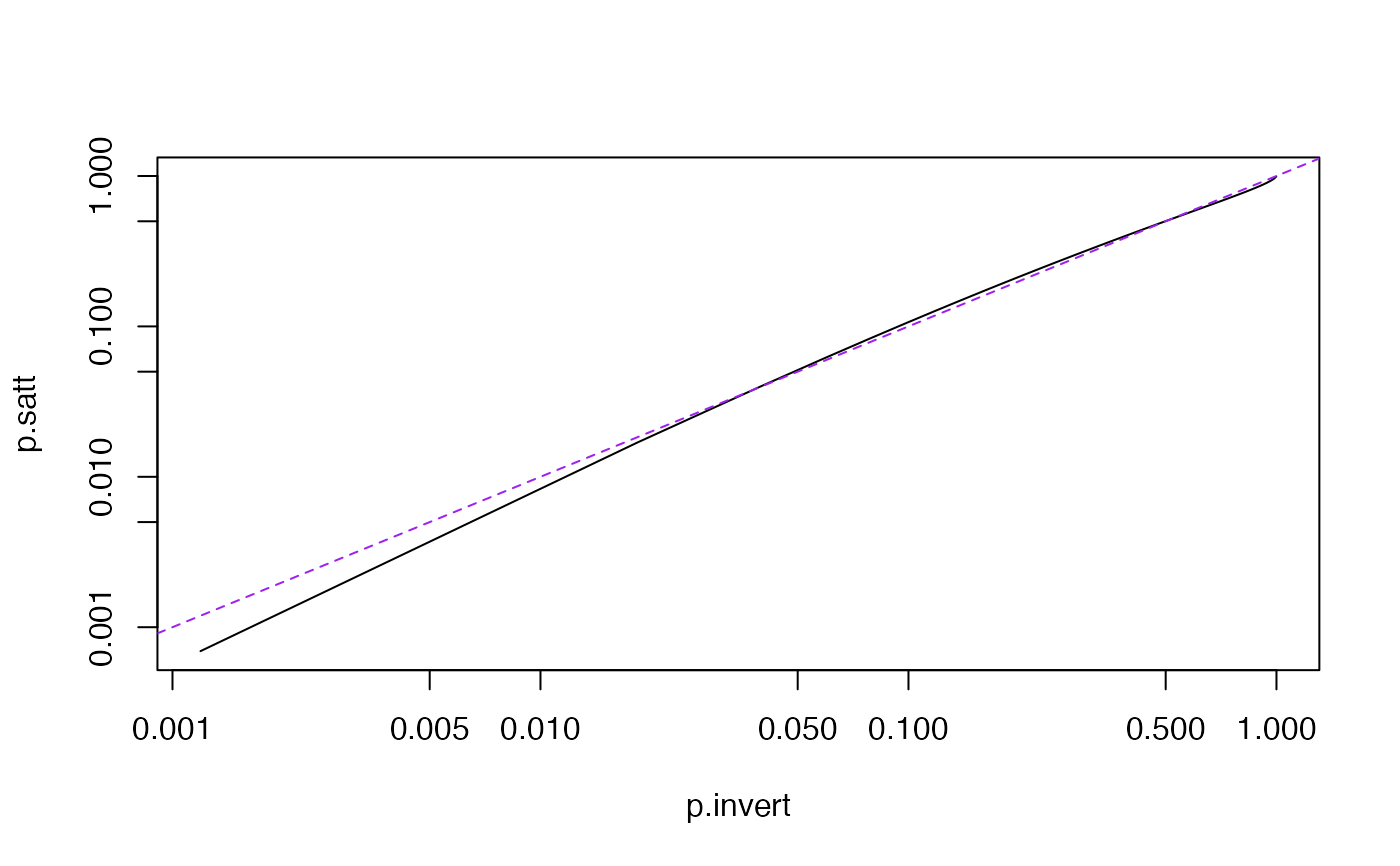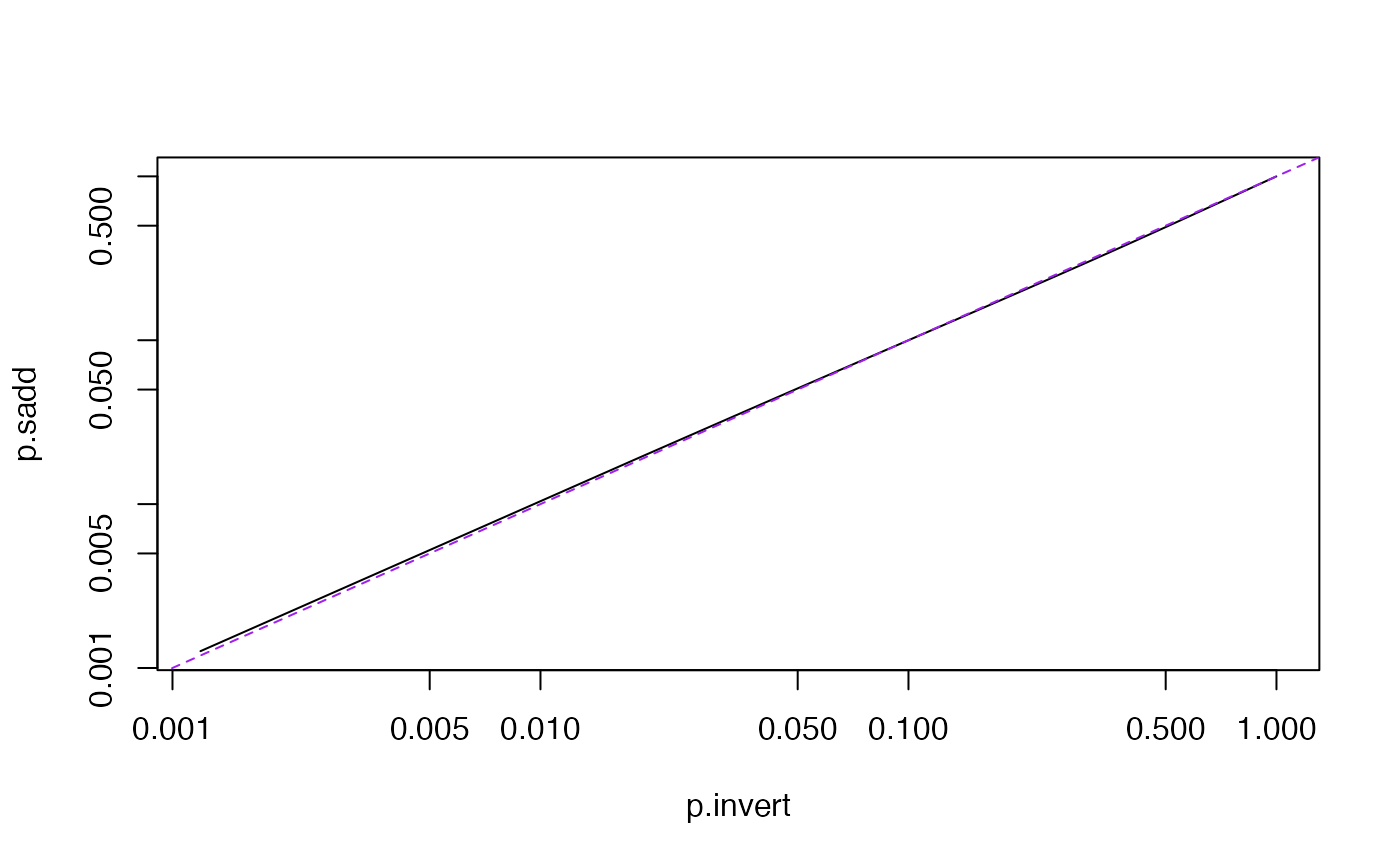The distribution of a quadratic form in p standard Normal variables is a linear combination of p chi-squared distributions with 1df. When there is uncertainty about the variance, a reasonable model for the distribution is a linear combination of F distributions with the same denominator.

pchisqsum(x, df, a, lower.tail = TRUE,
pFsum(x, df, a, ddf=Inf,lower.tail = TRUE,
method = c("saddlepoint","integration","satterthwaite"), ...)

## Arguments

x

Observed values

df

Vector of degrees of freedom

a

Vector of coefficients

ddf

Denominator degrees of freedom

lower.tail

lower or upper tail?

method

See Details below

...

arguments to pchisqsum

## Value

Vector of cumulative probabilities

## Details

The "satterthwaite" method uses Satterthwaite's approximation, and this is also used as a fallback for the other methods. The accuracy is usually good, but is more variable depending on a than the other methods and is anticonservative in the right tail (eg for upper tail probabilities less than 10^-5). The Satterthwaite approximation requires all a>0.

"integration" requires the CompQuadForm package. For pchisqsum it uses Farebrother's algorithm if all a>0. For pFsum or when some a<0 it inverts the characteristic function using the algorithm of Davies (1980). These algorithms are highly accurate for the lower tail probability, but they obtain the upper tail probability by subtraction from 1 and so fail completely when the upper tail probability is comparable to machine epsilon or smaller.

If the CompQuadForm package is not present, a warning is given and the saddlepoint approximation is used.

"saddlepoint" uses Kuonen's saddlepoint approximation. This is moderately accurate even very far out in the upper tail or with some a=0 and does not require any additional packages. The relative error in the right tail is uniformly bounded for all x and decreases as p increases. This method is implemented in pure R and so is slower than the "integration" method.

The distribution in pFsum is standardised so that a likelihood ratio test can use the same x value as in pchisqsum. That is, the linear combination of chi-squareds is multiplied by ddf and then divided by an independent chi-squared with ddf degrees of freedom.

pchisq

## Examples

x <- 2.7*rnorm(1001)^2+rnorm(1001)^2+0.3*rnorm(1001)^2
x.thin<-sort(x)[1+(0:50)*20]
p.invert<-pchisqsum(x.thin,df=c(1,1,1),a=c(2.7,1,.3),method="int" ,lower=FALSE)
p.satt<-pchisqsum(x.thin,df=c(1,1,1),a=c(2.7,1,.3),method="satt",lower=FALSE)

plot(p.invert, p.satt,type="l",log="xy")
abline(0,1,lty=2,col="purple")